Connect with us

# Revenue Recognition: Completed-Contract And Percentage-Of-Completion MethodPublished

on

In the construction industry, two accounting approaches have developed over the years regarding the recognition of revenue. The first approach—the completed-contract method—does not recognize any profit until the construction project is complete. The second approach—the percentage-of-completion method—recognizes profit on a piecemeal basis.

The logic behind the percentage-of-completion method is that both the buyer and seller have obtained enforceable rights. The buyer has the right to require specific performance on the contract; the seller has the right to require progress payments. Thus the facts seem to indicate that a continuous “sale” is in progress.

According to Statement of Position 81-1, the percentage method should be used if estimates of progress toward completion, revenues, and costs are reasonably dependable, and all the following conditions exist:

1. The contract clearly specifies the rights regarding goods or services to be provided, and the consideration to be exchanged.
2. The buyer can be expected to satisfy all the contractual obligations.
3. The contractor can be expected to perform the contractual obligations.

If these conditions have not been met, then the completed-contract method should be used. It should be emphasized that the total profit on the construction project is the same under both methods.

The difference between methods is simply a question of timing—the percentage method recognizes profit little by little over time, while the completed-contract method defers the entire profit until completion.

Let’s go in detail with case examples, its formula, calculation and journal entries……

The Completed-Contract

This method defers all the profit on the construction project until the completion date. During the construction period, all costs incurred are debited to an inventory account called Construction in Process“. This is similar to the Work in Process account used in cost accounting. Billings are debited to Accounts Receivable and credited to an account calledBillings on Construction“. This is not a revenue account since this method does not recognize any revenue or profit until completion. Rather, it is a contra asset to the Construction in Process Account. Finally, at completion, the construction and billings accounts are closed, and the difference between them is recognized as gross profit.

For easier understanding, let’s construct some examples…..

Example-1

Construction Corporation enters into a contract on January 1, 19A, with the Department of City Development to build a small building for \$100,000. The project is estimated to take 3 years. The following information presents the transactions that took place over this time: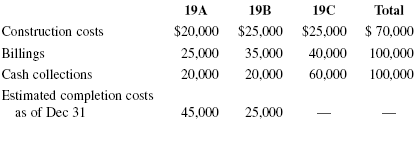Notice that the final profit is \$30,000 (\$100,000 ? \$70,000). Also notice that the estimate of what the final profit would be changed between 19A and 19B. In 19A it was \$35,000 (selling price of \$100,000 ? \$20,000 of costs incurred so far ? \$45,000 of estimated completion costs). In 19B it changed to \$30,000 (\$100,000 ? \$20,000 ? \$25,000 ? \$25,000). The journal entries for the three years would be as follows: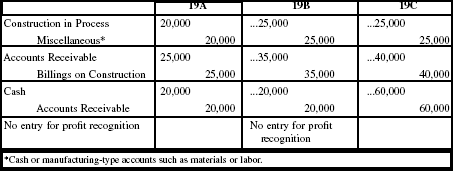By the end of 19C, the “billings account” and the “Construction in Process account” appear as follows: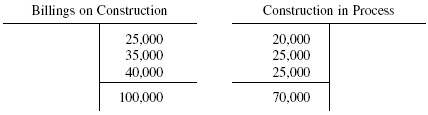The journal entry in 19C (to close these accounts and recognize profit) would be:

[Debit]. Billings on Construction = \$100,000
[Credit]. Construction in Process = \$70,000
[Credit]. Income on Construction = \$30,000

The information given regarding estimated completion costs was not needed in this problem. However, it is relevant if the percentage-of-completion method is used instead of the completed-contract method.

Notes: The account Billings on Construction is a contra to the construction account and is shown on the balance sheet as such. If its balance is less than the balance in the construction account, the net amount is shown as a current asset; if it is more, the net amount is shown as a current liability.

Example-2

In the previous example, the balance sheets for 19A and 19B would appear as follows: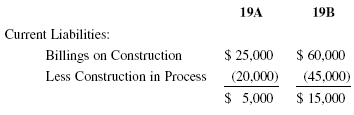Note: The income statements for 19A and 19B would not show any revenue or profit since these items are deferred until completion.

Example-3

If in 19X1 Corporation X has construction costs of \$50,000 and billings of \$38,000, its balance sheet would show: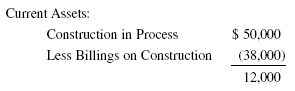The Percentage-Of-Completion Method

This method recognizes a portion of the gross profit each year based upon the following formula:

Recognized rofit: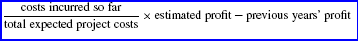The entries each year would be the same as under the completed-contract method, with one additional annual entry to recognize profit. This entry debits the construction account (the profit is placed “into” the inventory) and credits a profit account.

Example:

Let’s use the same information as in Example-1. The journal entry in 19A for profit recognition is:

[Debit]. Construction in Process = \$10,769
[Credit]. Income from Construction = \$10,769

The calculation is: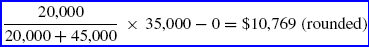At this point, the expected profit is \$35,000 (selling price of \$100,000 – past costs of \$20,000 – future costs of \$45,000).

The \$10,769 would be shown on the 19A income statement. In 19B the profit recognized is: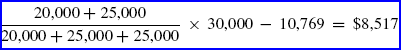Notice that the total expected profit has changed from \$35,000 to \$30,000 due to changes in anticipated costs. This happens often in the construction industry.

The \$30,000 total expected profit is computed as follows: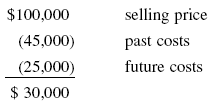The 19B income statement would show profit of \$8,517 and the journal entry would be:

[Debit]. Construction in Process = \$8,517
[Credit]. Income from Construction = \$8,517

While in 19C: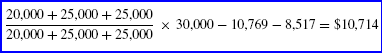A journal entry would be made for this amount. An examination of the accounts at the end of 19C reveals the following: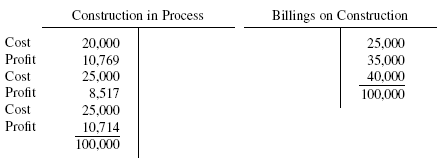Notice that the balances of these two accounts are equal (at \$100,000) under this method. This is because the construction account contains both cost and profit.

One final journal entry would be made at the end of 19C to close these two accounts:

[Debit]. Billings on Construction = \$100,000
[Credit]. Construction in Process = \$100,000

Note: If at any time during the construction period it is estimated that a loss will occur on the project (because the estimated total costs are expected to be higher than the selling price), it should be recognized by a debit to a loss account and a credit to the inventory account. Furthermore, if in previous years profit was recognized under the percentage-of completion method, it should now be nullified via a reversing entry.

Next Example:

A company used the completed-contract method for a 5-year construction project. Thus no profit would be recognized until the fifth year. During the third year the company realizes that the total project will result in a net loss of \$50,000. The company should immediately make the following journal entry:

[Debit]. Loss on Construction = \$50,000
[Credit]. Construction in Process = \$50,000

One Final Example:

In the previous example, assume the company used the percentage-of-completion method and recognized profit of \$10,000 and \$20,000, respectively, in the first 2 years. In the third year, upon discovery of the \$50,000 loss, an entry must be made to both recognize this loss, and to nullify the previous profit. The journal entry would be:

[Debit]. Loss on Construction = \$80,000 *
[Credit]. Construction in Process = \$80,000

Note: *10,000 + 20,000 + 50,000

Are you looking for easy accounting tutorial? Established since 2007, Accounting-Financial-Tax.com hosts more than 1300 articles (still growing), and has helped millions accounting student, teacher, junior accountants and small business owners, worldwide.